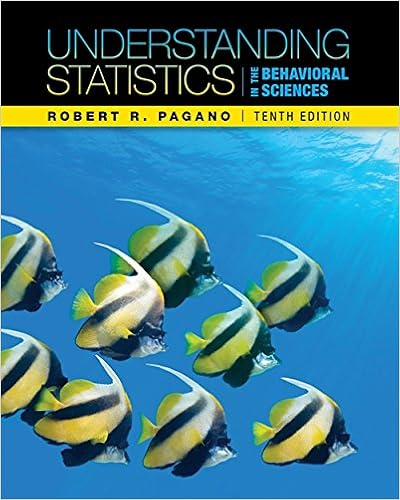# 8 jg problem 326 p 341 use spss this problem requires

• underwab
• 2

This preview shows page 1 - 2 out of 2 pages.

##### We have textbook solutions for you!
The document you are viewing contains questions related to this textbook.The document you are viewing contains questions related to this textbook.
Chapter 14 / Exercise 2-1
Understanding Statistics in the Behavioral Sciences
PaganoExpert Verified
8.JG Problem 3.26, p. 341. Use SPSS. This problem requires you to read a data file from the R Drive that is saved in the folder R:\STAT\Gabrosek Stephenson Materials\SPSS Datasets. The data file is named California Disabled. Problem 11 on this homework works with the same data set. Do those problems at the same time. 9.JG Problem 3.40, pp. 344-345A. That at least 50% of the subjects correctly identified 66.7% or more of the female samples. B. False1
##### We have textbook solutions for you!
The document you are viewing contains questions related to this textbook.The document you are viewing contains questions related to this textbook.
Chapter 14 / Exercise 2-1
Understanding Statistics in the Behavioral Sciences
PaganoExpert Verified
Homework #7Sections: 3.1-3.7STA 215 Abigail UnderwoodFall 2016
10. JG Problem 3.41, p. 345
11. JG Problem 3.43, p. 346. Use SPSS. This problem requires you to read a data file from the R Drive that is saved in the folder R:\STAT\Gabrosek Stephenson Materials\SPSS Datasets. The data file is named California Disabled. Problem 8 on this homework works with the same data set. Do those problems at the same time.
12. JG Problem 3.50, p. 349A. YesB. A= 0.270, B= 0.24, C= 0.30, D= 0.21, E= 0.33, F= 0.18, G= 0.36C. 68%D. 2.5%
2
•••